# Squaring Technique to speed up calculations

Many readers have requested me to teach them a simple method to square two and three digit numbers. In bank exams calculation speed is very crucial. So today I have decided to explain my Squaring technique.

## Technique

In case of two digit number deduct last digit and add it to another number and then add square of same.

In this technique we simplify the squaring method by making one unit's digit zero. It is far easy to multiply 50*24 than 54*24. So I used this technique. Try practice more to become expert in this technique.

## Let's take some examples

Find square of 53.
=(53*53)
= (53+3) * (53-3) + (3*3)
=(56*50) + 9
= (560*5) + 9
= 2800 + 9 = 2809

Let's take another example
Find square of 69
= (69*69)
= (69+1) * (69-1) + (1*1)
= (70*68) + 1
= (680*7) + 1
= 4761

Let's take one more example
Find square of 45
= (45*45)
= (45-5)*(45+5) + (5*5)
= (40*50) + 25
= 2000+25
= 2025

Comment below in case you find any problem in this technique.#### What's trending in BankExamsToday

Smart Prep Kit for Banking Exams by Ramandeep Singh - Download here1.hello sir....in 53 square u have taken 3*3 and in 69 square u have taken 1*1....can u tell me y u have taken and the trick?

1.=69*69
=(69-9)*(69+9)+(9*9)
=60*78+81
=(780*6)+81
=4680+81
=4761

2.(a^2 - b^2) = (a+b)(a-b)

a^2= (a+b)(a-b) + b^2

2.thanku sir.... :) ur website is very helpful .

1.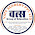hi

how find this study material

3.Yes use same technique

4.sir how to square more then 2 digits no?same techcnique will be implemented or not?

5.very good technique thanks sir

6.Hello Sir, You are doing very good :)

7.how to multiply with which no. how it will come to know

8.Wow, its really fantastic technique. .By using like this techniques we can surely increase our mathematical speed in any competitive exms. .thank you

9.why u r using 1 instead of 9 while squaring 69

1.you can use 9 also but its easy to use 1. Main idea is to come to tens digit by whatever addition or subtraction.

10.Sir is it necessary to mug up the sqiare roots till 30.

1.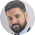Yes, it is required

11.Nice technique....

12.I know other technique bt from 26 to 75 squares for that 1 should know squares of 1 to 25, which i feel is better than this technique bcoz u dont need to multiply any but v should do addition. I'm taking the same examples given here

53 square = 2500+(100*number more than 50)+square of the increased number
i.e

IF THE NUMBER IS MORE THAN 50 DO SQUARES AS BELOW
Square of 53 = 2500 + 300 + 9 = 2809
Square of 69 = 2500 + 1900 + 361 = 4761

NOW IF THE NUMBER IS BELOW 50, DO AS BELOW
Square of 45 = 2500 - 500 + 25 = 2025

1.Your trick is really good. But while finding square of 83, I found it really long.

13.Square between 75-100
1.assume ,we have to find the square of N.first find the differencecwith 100 (lets say the difference isD)
2.find the square of D it will give us the last two digits if square
3.find N-D. It will give us the first two digits of the square
example:
Square of 93
100-93=7
7*7=49
93-7=86
So 8649
Collected from bank power app

14.its very nice trick i liked it

15.sir your shortcut tricks were quite good but i would like to know about short cuts for imperfect squares and cubes

16.its really nycccccccccc

17.jst i want 2 know how to find cubes in simple process

18.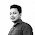Good techniques sir.keep it up..what is for 3 digits number??

19.CASE I

squaring any 2 digit no..
Eg. 83
split 83 to (8|3)^2
8^2=64 and 3^2 =9
8*3*2 =48

64|09
+4|8
____
6889

CASE II

Squaring any 3 digit no..
Split it into 2 parts
Eg.128
(12|8)^2

12^2=144
8^2=64
12*8*2=192

144|64
+19|2
_____
16384

CASE III

Squaring any number ending with 5
Eg. 85
Last 2 digit is 25
Remaining digit is 8*(8+1)
i.e) 8*9 =72

85^2= 7225

1.Really awesome thank you sir.....

2.Great shortcut... :)

3.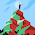20.Nice trick. great.....

21.Nice trick... Great. It make square calculation so easy.

22.I m giving one more trick may b some people will understand this method...
from number 35 to 75
1. Take value 25 for all squares between this range
2. Square of 48.
3. Take a mid 50 for all.
4. Calculate how less the value is less than 50 then minus it from 25. And the result is 23 and calculate the square of less value i.e. Square of 2 because value is 2 less from fifty the result is 04
5 merge these values result is 2304.

23.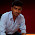my trick take 53
first take its square 2509 then 5*3=15*2=30 add it like this
2509
30
2809 simpleeeeeeeeeeeeeeeeeeeeeee

1.yes nice trick... I'm also posting the same trick here...
For 2 digit no, adding unitdigit with 10's
For 3 digit no, adding unitdigit with 100's
EG:1004 Split it into two parts : If second part has 2 digits then
10|06
100|0066
1|20
+_______
1012036

24.Squaring Technique for numbers having same digits...

>>111 Here there are 3 ones,
so write 123 and in reverse also 321. bt the middle term is common
12321

>>1111 Here 4 ones. 1234321

>>33 here 2 three's are there
3^2=9
write it as _0_9 (single digit gap b4 each digit)
Fill the first gap with next number of 0 i.e)1
Fill the second gap with previous number of 9 i.e) 8
ANS : 1089

>>333
write it as _ _ 0 _ _ 9
Ans : 1100889

Same technique can be applied for 6 and 9

>>666 (6^2 = 36)
write it as _ _ 3 _ _ 6
Ans : 443556

>>999 (9^2 = 81)
write it as _ _ 8 _ _ 1
Ans : 998001

25.Thanks I got easy technique

26.sir how can we do squaring of 4 digits like...1432 nd more than 4 digits like...5432819 etc with shortcuts ?

27.hello sir how can we find out the sqaure of 1432 with shortcuts nd more than 4 digits like 73214,5432819 etc ?

28.45*45 this another simple way is take last digit multiply 5*5=25....................then take 4 next number of 4 is 5 so multiply both we get 4*5 =20 so 45*45= 2025

29.please tell the method of squaring 149*149

1.(150-1)*(150-1)=22500+1-2(150)=22201

30.149*149 => FIRST ADD 149 WITH 49 OF SECOND DIGIT 149+49=198 THIS IS OUR FIRST PART ANSWER FOR SECOND PART JUST MULTIPLY 49 OF FIRST ONE WITH 49 OF SECOND ONE =49*49 NOW WE HAVE ALREADY GET FIRST PART NOW SECOND PART JUST ADD BOTH OF THEN AS

198
2401
22201

31.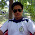hello sir....in 53 square u have taken 3*3 and in 69 square u have taken 1*1....can u tell me y u have taken and the trick?

32.1.by using this formula ,u can find out square of any number.
square of 45=?
a=4,b=5
use it a^2+2ab+b^2 ,put last digit only and remaining will be remainder.
2025

33.34.